# Hours and days

## Learning focus

Learn about the number of minutes in an hour and the number of hours in a day.

This lesson includes:

• one learning summary
• two interactive activities

# Learn

In this lesson, you will learn how we measure time on a clock and estimate how long different events take.

First, look at the slideshow below to understand how we measure time using clocks. Try looking at a clock or watch in your home to help you to answer the questions.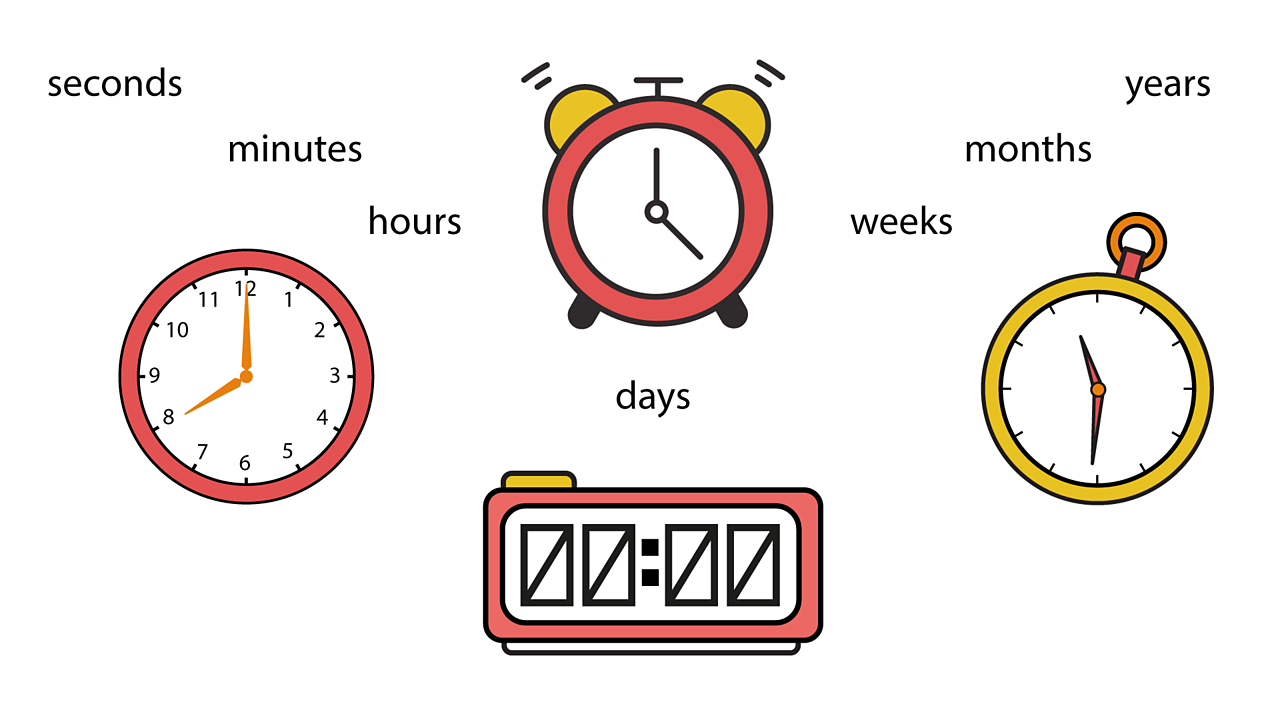Time is a unit that can be measured. We use different instruments to measure time. We measure it in different units such as seconds, minutes, hours, days, weeks, months and years.1 of 5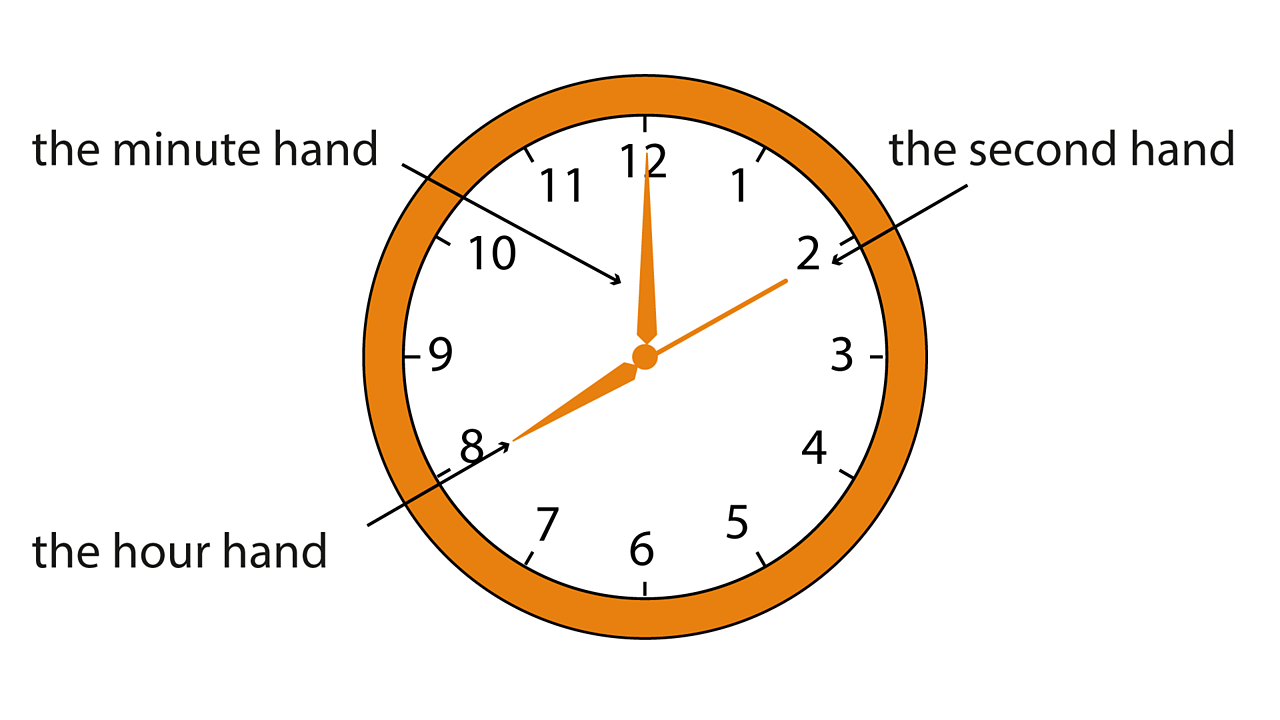We can use a clock to measure time. On a clock there is a minute hand, an hour hand and sometimes a second hand. So we can measure seconds, minutes and hours.2 of 5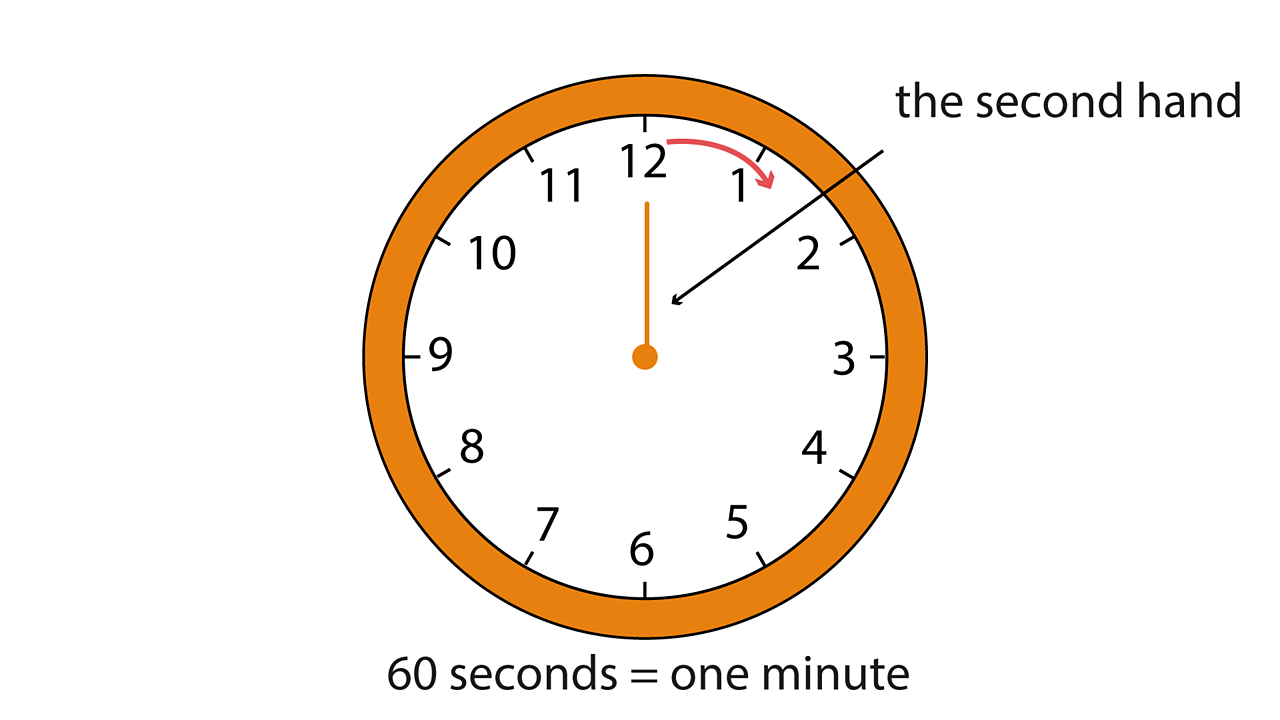This shows the second hand on a clock. How many seconds are in a minute? There are 60 seconds in a minute.3 of 5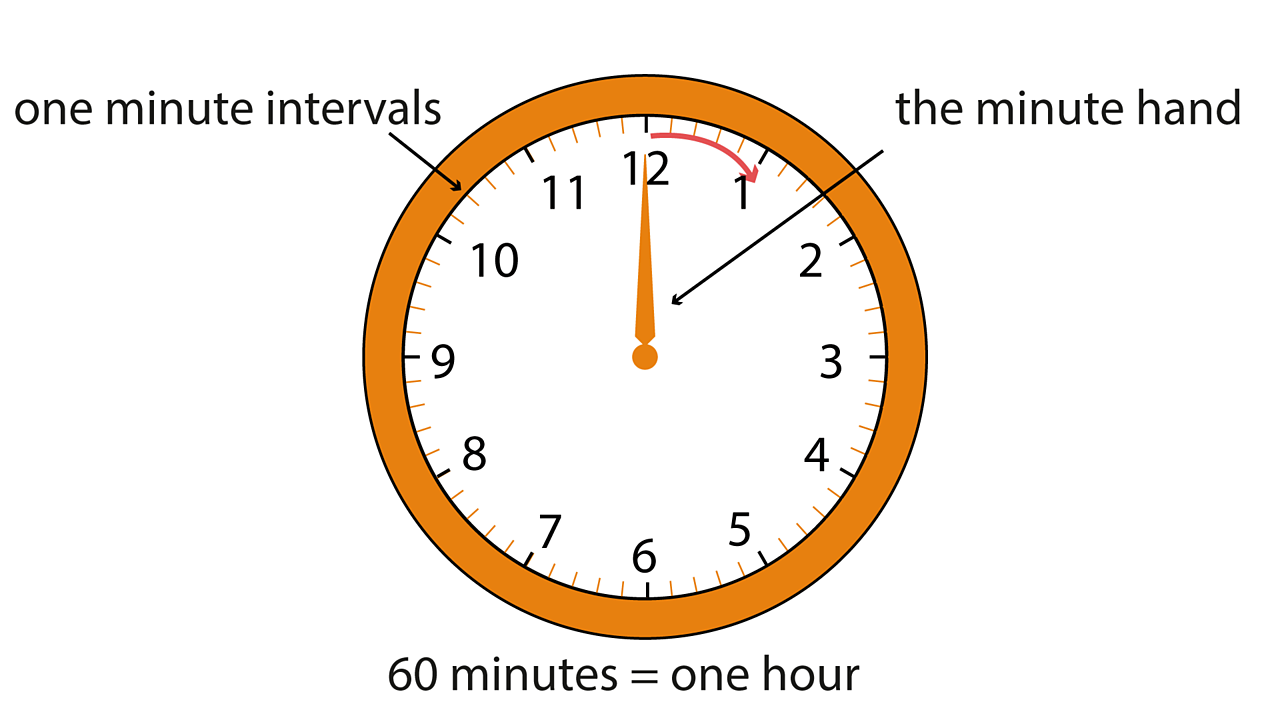This shows the minute hand on a clock. How many minutes are in an hour? There are 60 minutes in an hour.4 of 5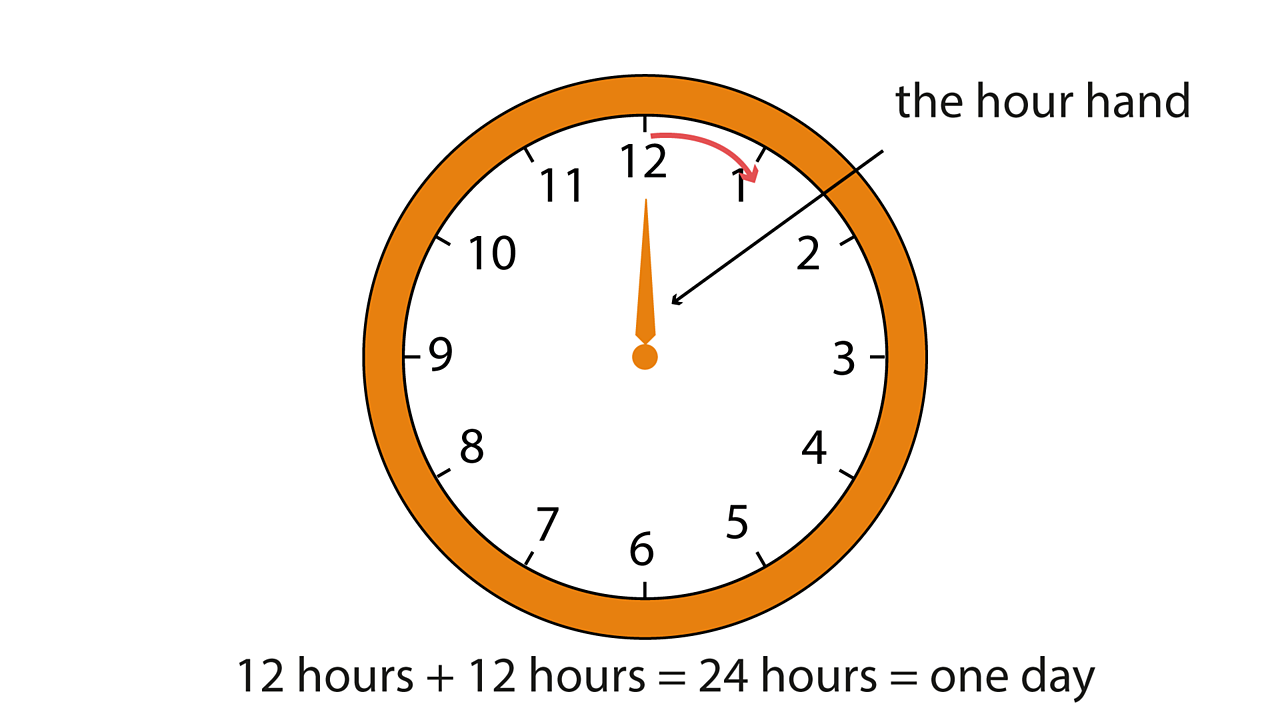This shows the hour hand on a clock. How many hour intervals are there on the clock? There are 12. The hour hand goes round the clock twice in a day. So 12 hours + 12 hours = 24 hours or 1 day!5 of 5

### Just a minute

You can use words to help count a minute without using a stopwatch. You can say '1 elephant, 2 elephants, 3 elephants' and so on for each second until you get to 60 elephants for 1 minute.

Can you think of any other words that you could use to help you to count to a minute?

Now let's look at some more units of time on a clock in the next slideshow.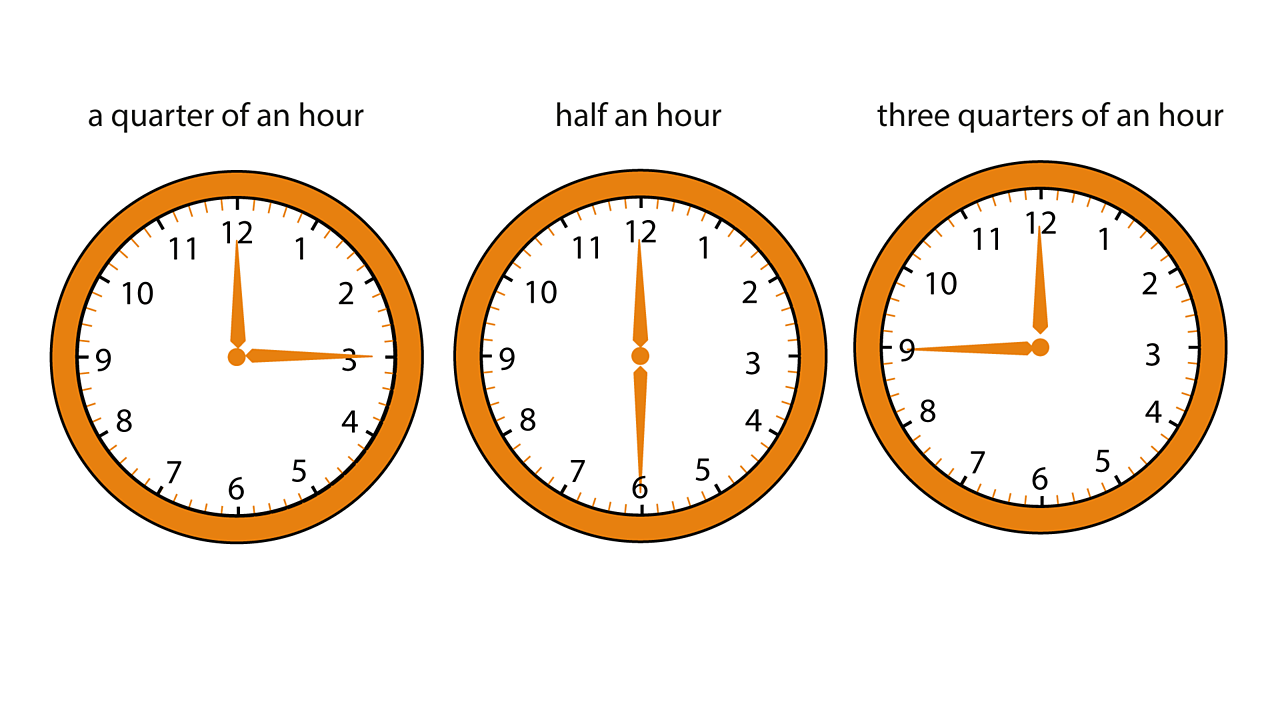How many minutes are there in a quarter of an hour? How many minutes are there in half an hour? How many minutes are there in three quarters of an hour?1 of 4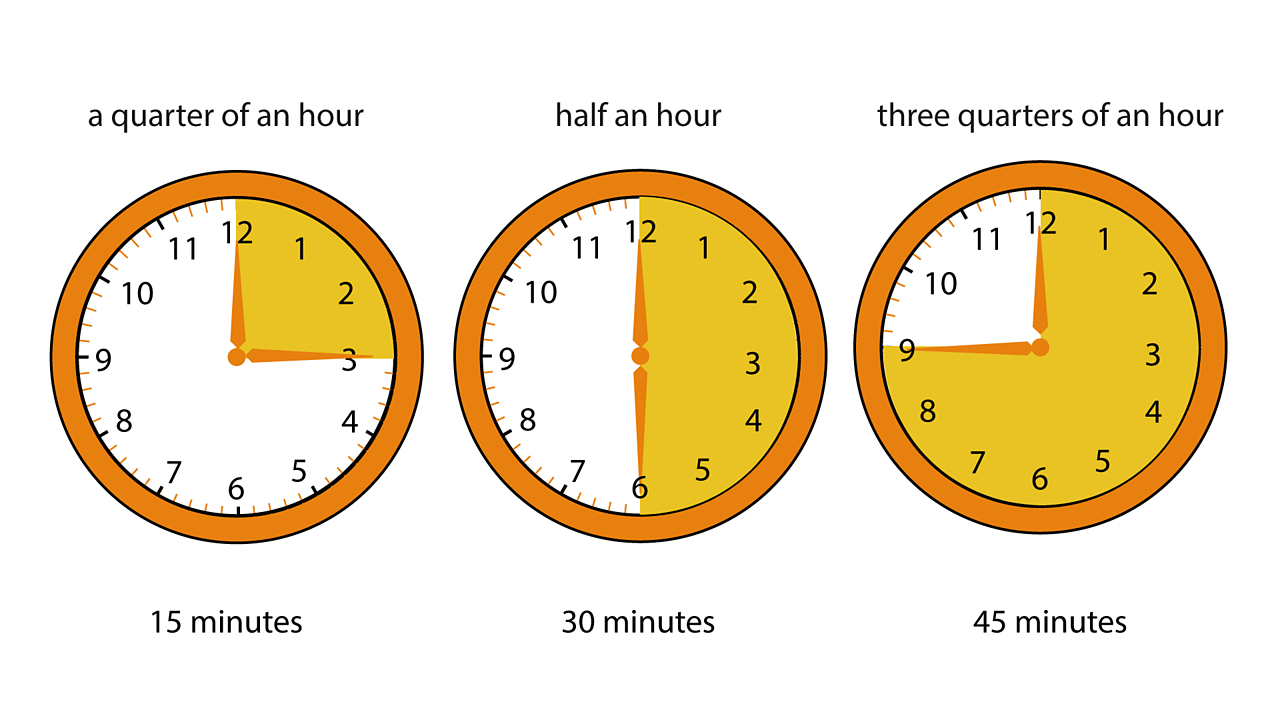There are 15 minutes in a quarter of an hour. There are 30 minutes in half an hour. There are 45 minutes in three quarters of an hour.2 of 4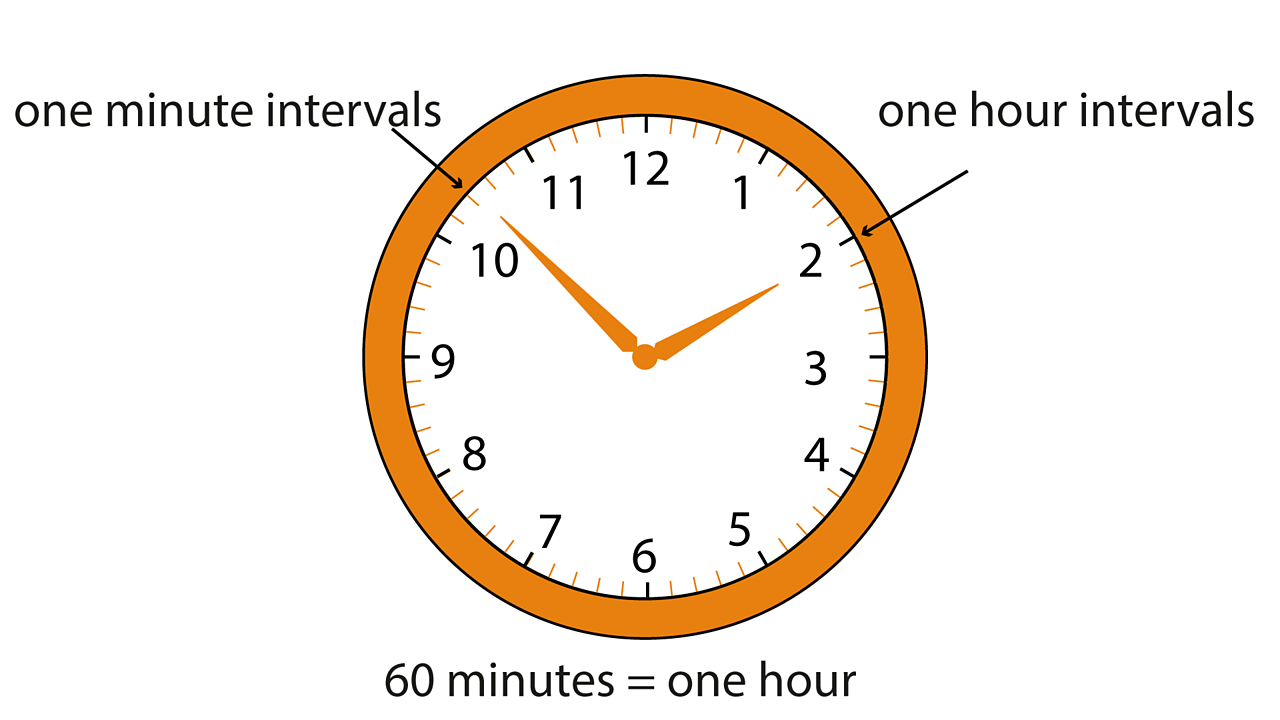How many minute intervals are there on the clock? How many one hour intervals are there?3 of 4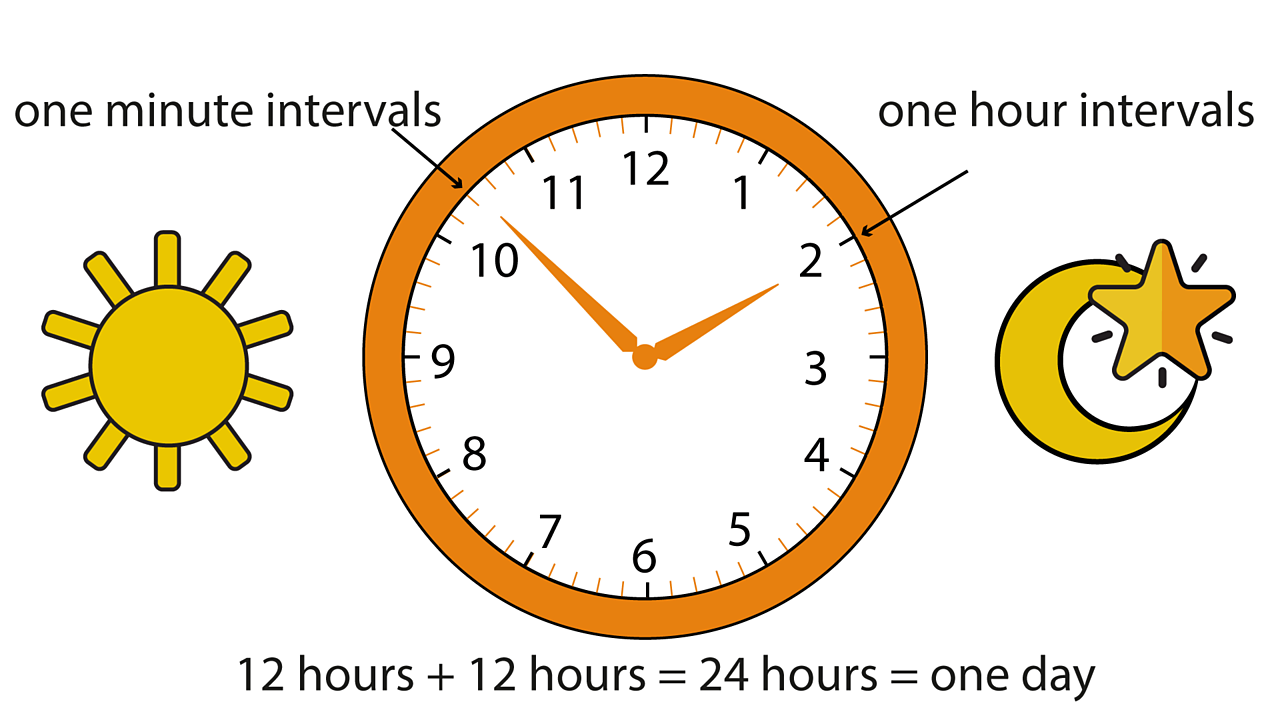There are 60 minute intervals and there are 12 one hour intervals. The hour hand goes round the clock twice. 12 hours + 12 hours = 24 hours = 1 day.4 of 4

# Practise

## Activity 1

Time to put your knowledge to the test! Here is a quiz all about minutes in an hour and hours in a day.

## Activity 2

Now try and solve some problems using what you have learned about hours and days.

# There's more to learn

Have a look at these other resources around the BBC and the web.